# How to Calculate and Solve for the Current, Time and Quantity of Charge | Nickzom Calculator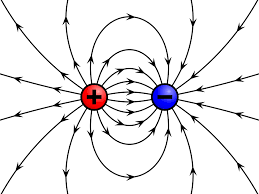The image above represents the quantity of charge.

To compute the quantity of charge, two essential parameters are needed and the parameters are current (I) and time (t).

The formula for calculating the quantity of charge;

Q = It

Where;
Q = Quantity of Charge
I = Current
t = Time

Let’s solve an example;
Find the quantity of charge when the current (I) is 24 amp with a time of 8 secs.

This implies that;
I = Current = 24 amp
t = Time = 8 secs

Q = It
Q = 24 x 8
Q = 192

Therefore, the quantity of charge is 192 coulombs (C).

Calculating the Current (I) of a charge using the Quantity of Charge (Q) and the time (t).

I = Q / t

Where;
I = Current
Q = Quantity of the charge
t = Time

Let’s solve an example;
Find the current of a charge with the quantity of the charge as 150 coulombs (C) and time as 15 secs.

This implies that;
Q = Quantity of the charge = 150 C
t = Time = 15 secs

I = Q / t
I = 150 / 15
I = 10

Therefore. the current is 10 Ampere (A).

Calculating the Time (t) of a charge using the Quantity of the charge (Q) and the Current (I).

t = Q / I

Where;
t = Time
Q = Quantity of charge
I = Current

Let’s solve an example;
Find the time of a charge when the quantity of the charge is 250 C with a current of 18 A.

This implies that;
Q = Quantity of charge = 250
I = Current = 18

t = Q / I
t = 200 / 18
t = 11.1

Therefore, the time is 11.1 secs.

Nickzom Calculator – The Calculator Encyclopedia is capable of calculating the quantity of charge.

To get the answer and workings of the quantity of charge using the Nickzom Calculator – The Calculator Encyclopedia. First, you need to obtain the app.

You can get this app via any of these means:

To get access to the professional version via web, you need to register and subscribe for NGN 1,500 per annum to have utter access to all functionalities.
You can also try the demo version via https://www.nickzom.org/calculator

Once, you have obtained the calculator encyclopedia app, proceed to the Calculator Map, then click on Electrolysis under the Physics section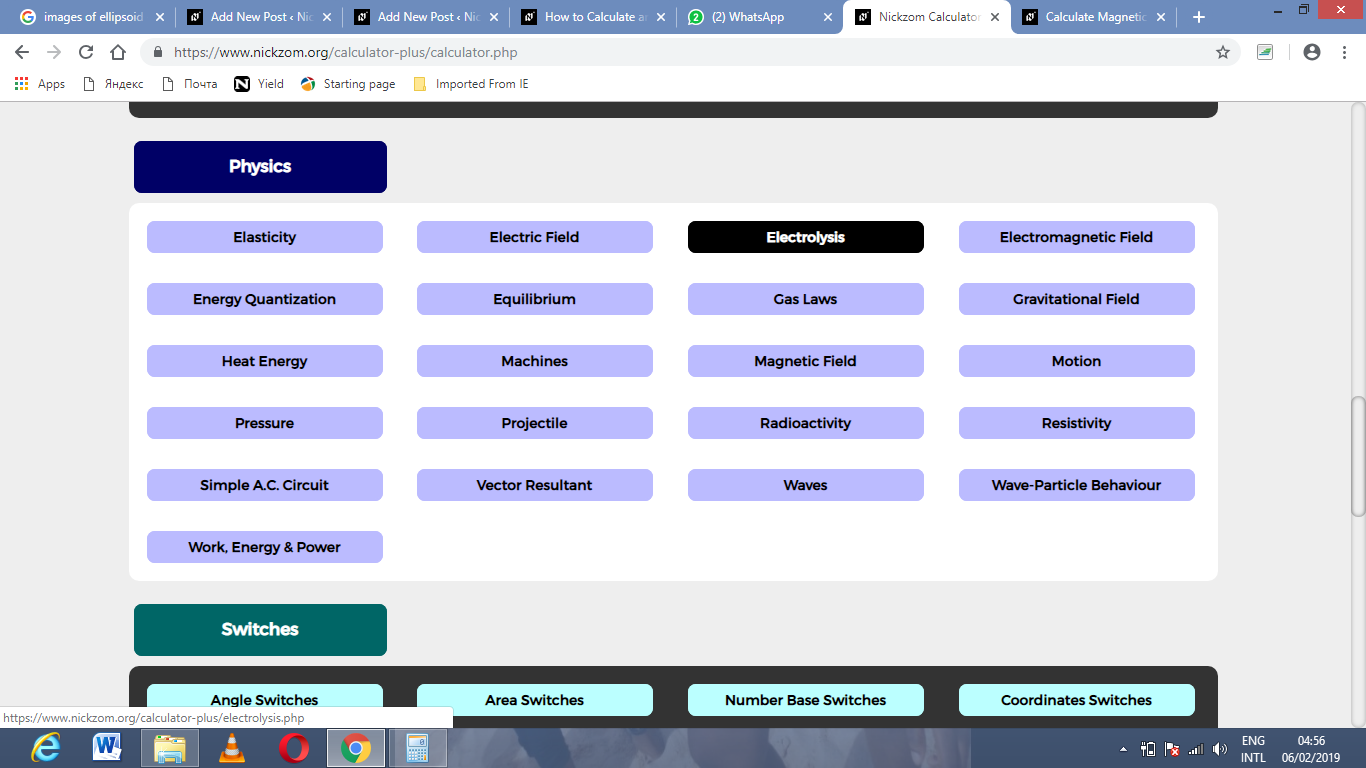Now, Click on Quantity of Charge under Electrolysis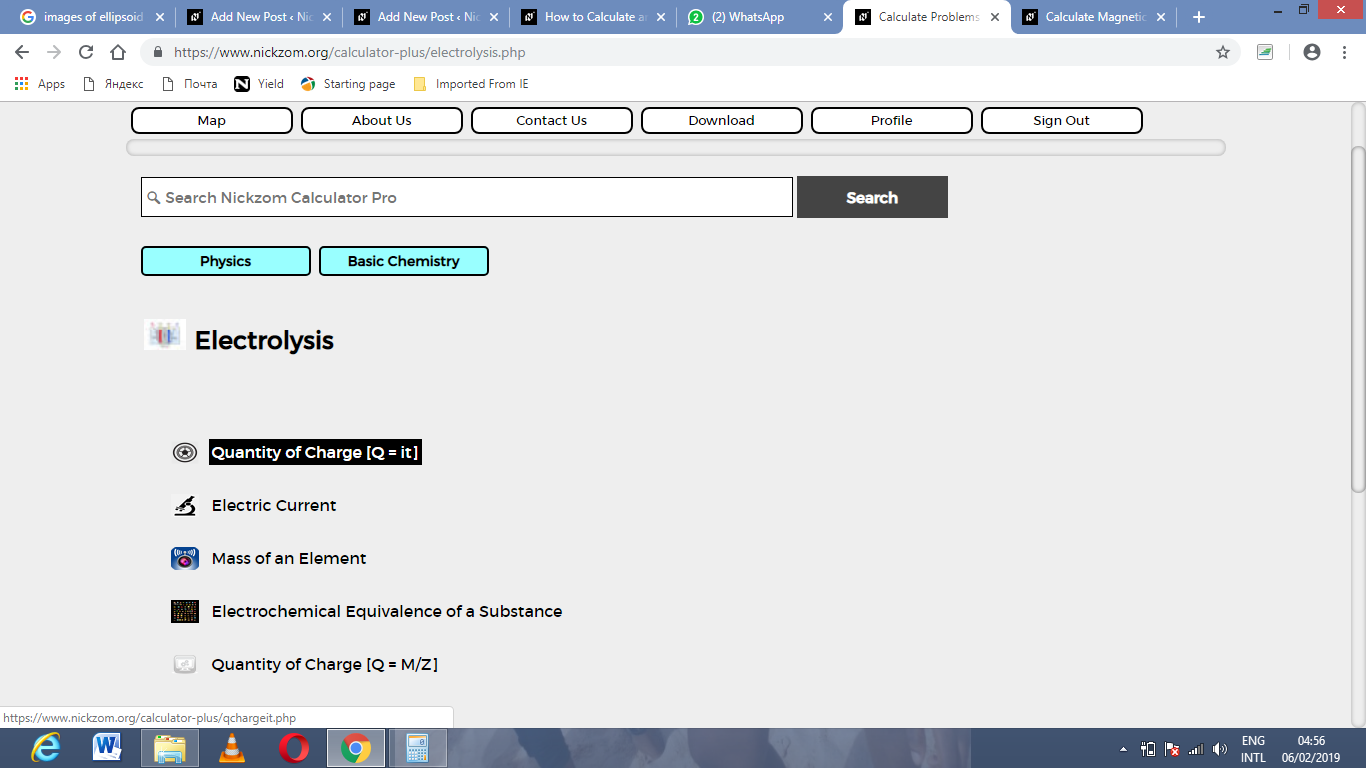The screenshot below displays the page or activity to enter your values, to get the answer for the quantity of charge according to the respective parameters which are the current (I) and time (t).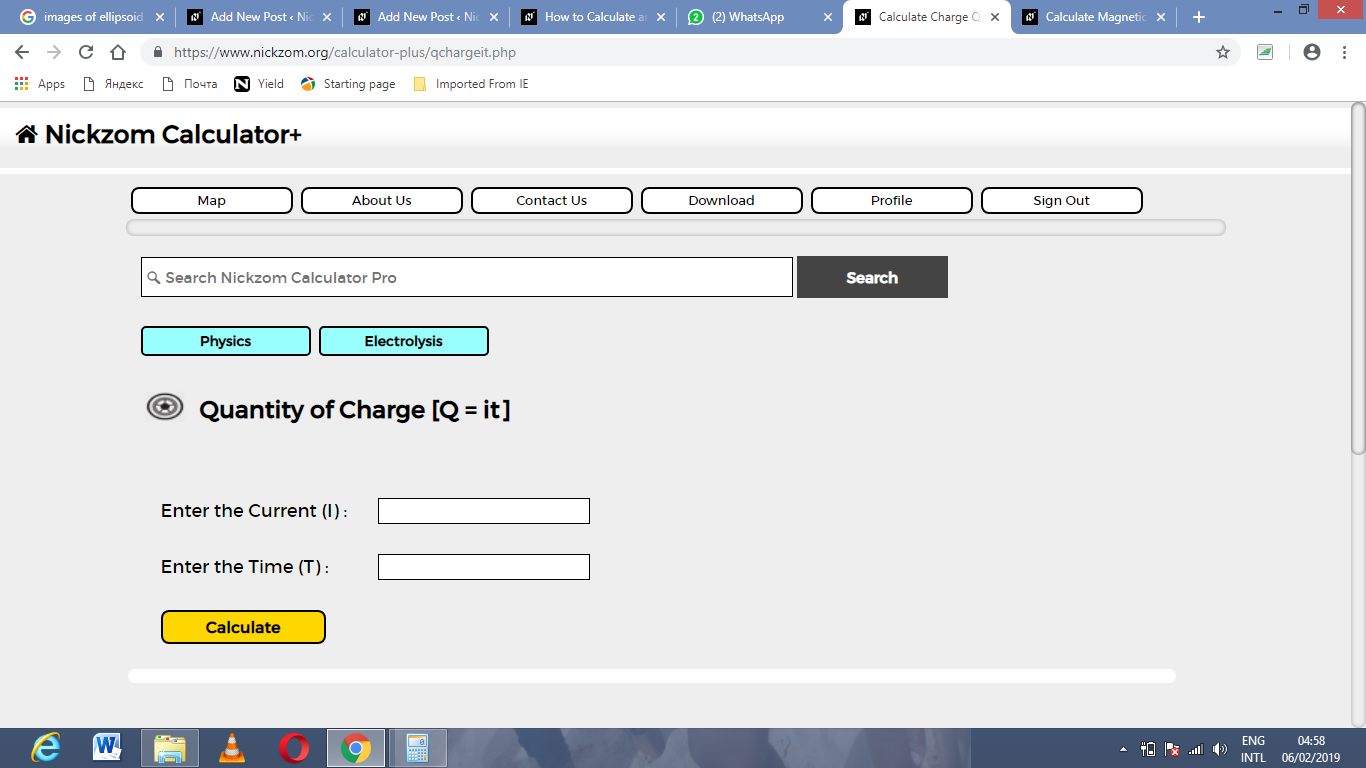Now, enter the values appropriately and accordingly for the parameters as required by the example above where the current (I) is 24 and time (t) is 8.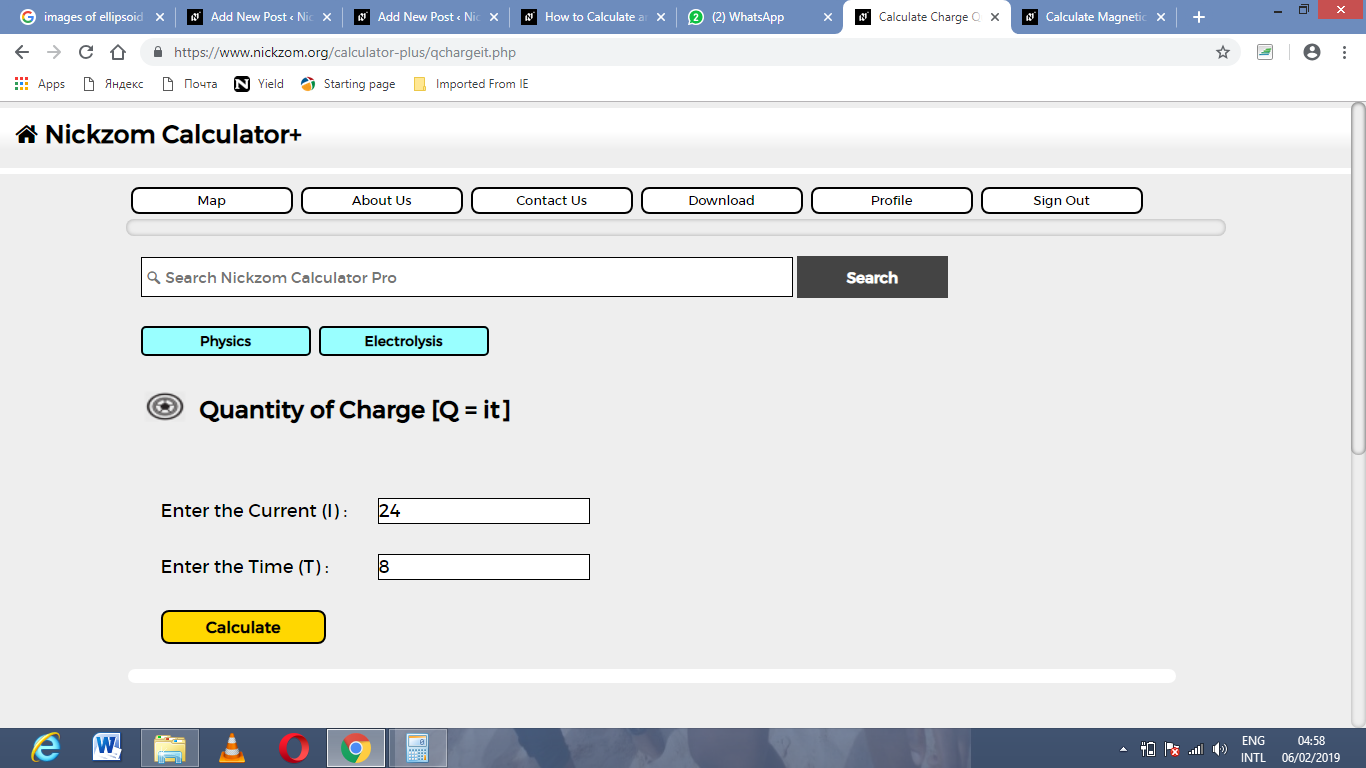Finally, Click on Calculate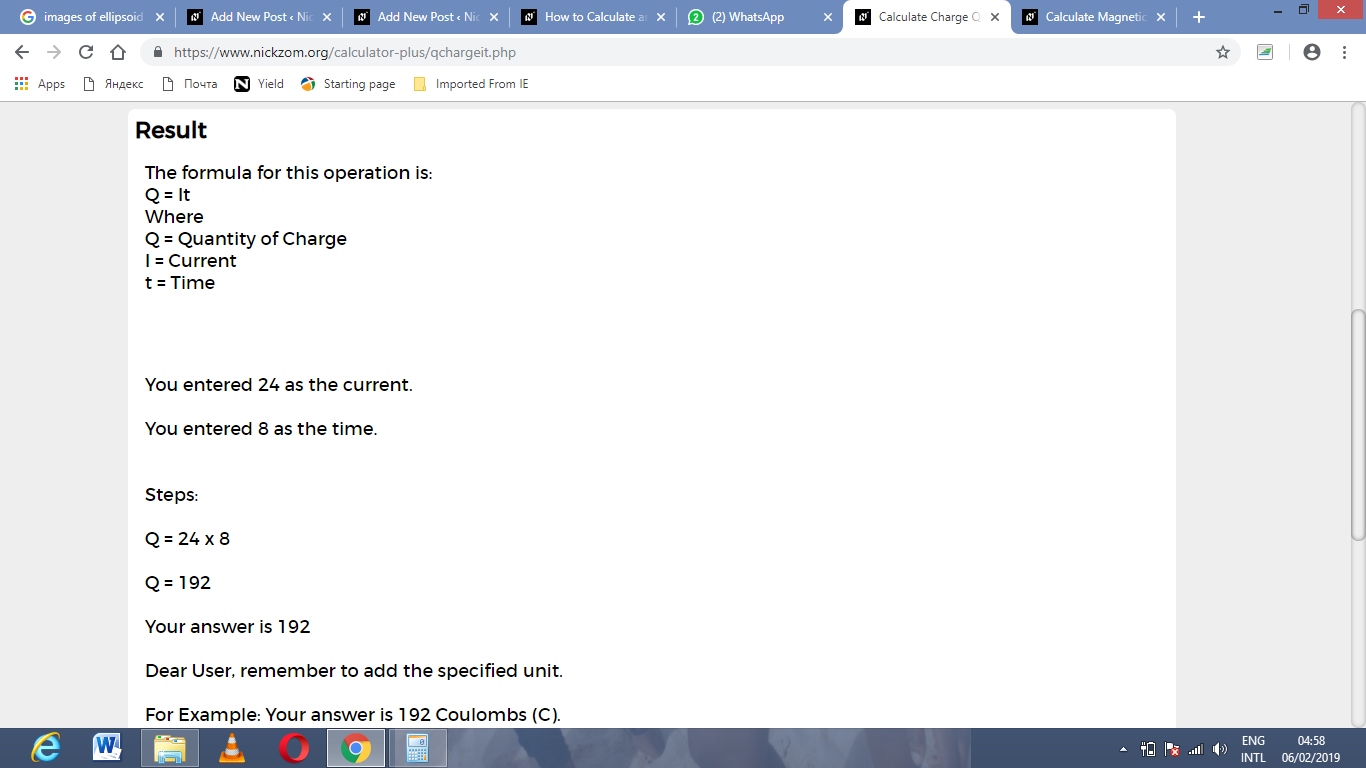As you can see from the screenshot above, Nickzom Calculator – The Calculator Encyclopedia solves for the quantity of charge and presents the formula, workings and steps too.